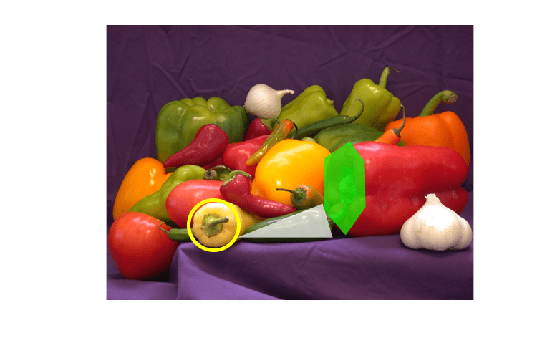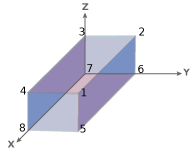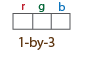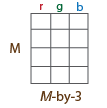# insertShape

Insert shapes in image or video

## Syntax

``RGB = insertShape(I,shape,position)``
``RGB = insertShape(___,Name=Value)``

## Description

example

````RGB = insertShape(I,shape,position)` returns a truecolor image with `shape` inserted. The input image, `I`, can be either a truecolor or grayscale image. You draw the shapes by overwriting pixel values.```

example

````RGB = insertShape(___,Name=Value)` specifies options using one or more name-value arguments in addition to the previous syntax. For example,`insertShape(I,"circle",[150 280 35],LineWidth=5)` sets the line width value to `5`.```

## Examples

collapse all

Read an image into the workspace.

`I = imread("peppers.png");`

Draw a circle on the image with a border line width of 5.

`RGB = insertShape(I,"circle",[150 280 35],LineWidth=5);`

Draw a filled triangle and a filled hexagon on the image.

```pos_triangle = [183 297 302 250 316 297]; pos_hexagon = [340 163 305 186 303 257 334 294 362 255 361 191]; RGB = insertShape(RGB,"filled-polygon",{pos_triangle,pos_hexagon},... Color=["white","green"],Opacity=0.7);```

Display the resulting image.

`imshow(RGB);`## Input Arguments

collapse all

Input image, specified in truecolor or 2-D grayscale.

Data Types: `single` | `double` | `int16` | `uint8` | `uint16`

Type of shape, specified as `"rectangle"`, `"filled-rectangle"`, `"line"`, `"polygon"`, `"filled-polygon"`, `"circle"`, or `"filled-circle"`, `"projected-cuboid"`.

Data Types: `char`

Position of shape, specified according to the type of shape, described in the table.

ShapePositionExample
`"rectangle"`
`"filled-rectangle"`
For one or more rectangles, specify M-by-4 matrix where each row specifies a rectangle as $\left[\begin{array}{cccc}x& y& width& height\end{array}\right]$.

`$\left[\begin{array}{cccc}{x}_{1}& {y}_{1}& widt{h}_{1}& heigh{t}_{1}\\ {x}_{2}& {y}_{2}& widt{h}_{2}& heigh{t}_{2}\\ ⋮& ⋮& ⋮& ⋮\\ {x}_{M}& {y}_{M}& widt{h}_{M}& heigh{t}_{M}\end{array}\right]$`

Two rectangles, M=2`"line"`

`"polygon"`

`"filled-polygon"`

For one or more disconnected lines, specify an M-by-4 matrix, where each four-element vector, $\left[{x}_{1}\text{\hspace{0.17em}}{y}_{1\text{\hspace{0.17em}}}{x}_{2\text{\hspace{0.17em}}}{y}_{2}\right]$, describes a line with endpoints.

`$\left[\begin{array}{cccc}{x}_{11}& {y}_{11}& {x}_{12}& {y}_{12}\\ {x}_{21}& {y}_{21}& {x}_{22}& {y}_{22}\\ ⋮& ⋮& ⋮& ⋮\\ {x}_{M1}& {y}_{M1}& {x}_{M2}& {x}_{M2}\end{array}\right]$`

The polyline always contains (L-1) number of segments because the first and last vertex points do not connect.

Two lines, M=2For one or more polylines or polygons with the same number of vertices, specify an M-by-2L matrix, where each row is a vector, $\left[{x}_{1}\text{\hspace{0.17em}}{y}_{1\text{\hspace{0.17em}}}{x}_{2\text{\hspace{0.17em}}}{y}_{2}\text{\hspace{0.17em}}...\text{\hspace{0.17em}}{x}_{L}\text{\hspace{0.17em}}{y}_{L}\right]$, of consecutive vertex locations, representing a polyline or a polygon with L number of vertices.

`$\left[\begin{array}{ccccccc}{x}_{11}& {y}_{11}& {x}_{12}& {y}_{12}& \cdots & {x}_{1L}& {y}_{1L}\\ {x}_{21}& {y}_{21}& {x}_{22}& {y}_{22}& \cdots & {x}_{2L}& {y}_{2L}\\ ⋮& ⋮& ⋮& ⋮& \ddots & ⋮& ⋮\\ {x}_{M1}& {y}_{M1}& {x}_{M2}& {y}_{M2}& \cdots & {x}_{ML}& {y}_{ML}\end{array}\right]$`

Two polygons with equal number of vertices, M=2, L=5For one or more polylines or polygons with unequal number of vertices, specify an M-by-1 cell array, where each cell contains an L-by-2 matrix of [x,y] vertices, or a 1-by-2L vector, $\left[{x}_{1}\text{\hspace{0.17em}}{y}_{1\text{\hspace{0.17em}}}{x}_{2\text{\hspace{0.17em}}}{y}_{2}\text{\hspace{0.17em}}...\text{\hspace{0.17em}}{x}_{L}\text{\hspace{0.17em}}{y}_{L}\right]$, of consecutive vertex locations.

The value of L can be different for each cell element. For example,

`$\left\{\left[{x}_{1}\text{\hspace{0.17em}}{y}_{1\text{\hspace{0.17em}}}{x}_{2\text{\hspace{0.17em}}}{y}_{2}\right],\text{\hspace{0.17em}}\left[{x}_{1}\text{\hspace{0.17em}}{y}_{1\text{\hspace{0.17em}}}{x}_{2\text{\hspace{0.17em}}}{y}_{2}\text{\hspace{0.17em}}{x}_{3}\text{\hspace{0.17em}}{y}_{3}\right]\right\}$`

Two polygons with unequal number of vertices, M=2`"circle"`
`"filled-circle"`
An M-by-3 matrix, where each row is a vector specifying a circle as $\left[\begin{array}{ccc}x& y& radius\end{array}\right]$. The $\left[\begin{array}{cc}x& y\end{array}\right]$ coordinates represent the center of the circle.

`$\left[\begin{array}{ccc}{x}_{1}& {y}_{1}& radiu{s}_{1}\\ {x}_{2}& {y}_{2}& radiu{s}_{2}\\ ⋮& ⋮& ⋮\\ {x}_{M}& {y}_{M}& radiu{s}_{M}\end{array}\right]$``"projected-cuboid"`

An 8-by-2-by-M array or an M-by-8 matrix, where M specifies a projected cuboid.

When specified as an 8-by-2-M array, each row must contain the $\left[\begin{array}{cc}x& y\end{array}\right]$ location of a projected cuboid vertex. The vertices are connected to form a cuboid with six faces. The order of the input vertices must match the order shown in the diagram.

When specified as an M-by-8 matrix, each row specifies the front-facing and rear-facing sides of a projected cuboid in the form,

`$\left[\begin{array}{cccccccc}x1& y1& w1& h1& x2& y2& w2& h2\end{array}\right]$`

where, [x1 y1] and [x2 y2] specify the upper-left coordinates of the front-facing and back-facing sides, respectively. [w1 h1] and [w2 h2] specify the corresponding width and height.Data Types: `single` | `double` | `int8` | `int16` | `int32` | `int64` | `uint8` | `uint16` | `uint32` | `uint64`

### Name-Value Arguments

Specify optional pairs of arguments as `Name1=Value1,...,NameN=ValueN`, where `Name` is the argument name and `Value` is the corresponding value. Name-value arguments must appear after other arguments, but the order of the pairs does not matter.

Before R2021a, use commas to separate each name and value, and enclose `Name` in quotes.

Example: `Color="yellow"` sets the shape color to yellow.

Shape border line width, specified in pixels, as a positive scalar integer. This property only applies to the `"Rectangle"`, `"Line"`, `"Polygon"`, or `"Circle"` shapes.

Data Types: `uint8` | `uint16` | `int16` | `double` | `single`

Shape color, specified as a character vector, cell array of character vectors, vector, or an M-by-3 matrix. You can specify a different color for each shape or one color for all shapes. Color values must be specified in the range `[0,255]`. Values that have a range of `[0,1]` must be scaled by a value of 255 before using it with this function. For example, `[255 255 255].*colorvalue`.

Supported colors are: `"blue"`, `"green"`, `"red"`, `"cyan"`, `"magenta"`, `"yellow"`,`"black"`, and `"white"`.

ColorFormatExample
Specify one color for all shapes

String or character color name

`"r"`

`"red"`

1-by-3 vector (RGB triplet)

`[255 0 0]`Specify a color for each shapeM-element vector

`["red","yellow","blue"]`

M-by-3 matrix, as a list of RGB values

```255 0 0 255 0 0 0 255 255```Data Types: `cell` | `char` | `uint8` | `uint16` | `int16` | `double` | `single`

Opacity of filled shape, specified as a scalar value in the range [0 1]. The `Opacity` property applies to the `"filled-rectangle"`, `"filled-polygon"`, and the `"filled-circle"` shapes.

Data Types: `double` | `single` | `int8` | `int16` | `int32` | `int64` | `uint8` | `uint16` | `uint32` | `uint64`

Smooth shape edges, specified as a logical value of `1` (`true`) or `0` (`false`). A `true` value enables an anti-aliasing filter to smooth shape edges. This value applies only to nonrectangular shapes. Enabling anti-aliasing requires additional time to draw the shapes.

Data Types: `logical`

## Output Arguments

collapse all

Output image, returned as a truecolor image.

## Version History

Introduced in R2014a

expand all